# 15.2 Lewis acids and bases  (Page 4/12)

 Page 4 / 12

## Chemistry end of chapter exercises

Under what circumstances, if any, does a sample of solid AgCl completely dissolve in pure water?

when the amount of solid is so small that a saturated solution is not produced

Explain why the addition of NH 3 or HNO 3 to a saturated solution of Ag 2 CO 3 in contact with solid Ag 2 CO 3 increases the solubility of the solid.

Calculate the cadmium ion concentration, [Cd 2+ ], in a solution prepared by mixing 0.100 L of 0.0100 M Cd(NO 3 ) 2 with 1.150 L of 0.100 NH 3 ( aq ).

8 $×$ 10 –5 M

Explain why addition of NH 3 or HNO 3 to a saturated solution of Cu(OH) 2 in contact with solid Cu(OH) 2 increases the solubility of the solid.

Sometimes equilibria for complex ions are described in terms of dissociation constants, K d . For the complex ion ${\text{AlF}}_{6}{}^{\text{3−}}$ the dissociation reaction is:

${\text{AlF}}_{6}{}^{\text{3−}}⇌{\text{Al}}^{\text{3+}}+6{\text{F}}^{\text{−}}$ and ${K}_{\text{d}}=\frac{\left[{\text{Al}}^{\text{3+}}\right]{\left[{\text{F}}^{\text{−}}\right]}^{6}}{\left[{\text{AlF}}_{6}{}^{\text{3−}}\right]}=2\phantom{\rule{0.2em}{0ex}}×\phantom{\rule{0.2em}{0ex}}{10}^{-24}$

Calculate the value of the formation constant, K f , for ${\text{AlF}}_{\text{6}}{}^{\text{3−}}.$

5 $×$ 10 23

Using the value of the formation constant for the complex ion $\text{Co}{\left({\text{NH}}_{3}\right)}_{6}{}^{\text{2+}},$ calculate the dissociation constant.

Using the dissociation constant, K d = 7.8 $×$ 10 –18 , calculate the equilibrium concentrations of Cd 2+ and CN in a 0.250- M solution of $\text{Cd}{\left(\text{CN}\right)}_{4}{}^{\text{2−}}.$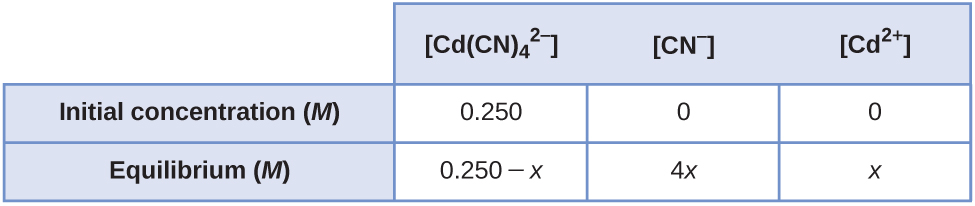[Cd 2+ ] = 9.5 $×$ 10 –5 M ; [CN ] = 3.8 $×$ 10 –4 M

Using the dissociation constant, K d = 3.4 $×$ 10 –15 , calculate the equilibrium concentrations of Zn 2+ and OH in a 0.0465- M solution of $\text{Zn}{\left(\text{OH}\right)}_{4}{}^{\text{2−}}.$

Using the dissociation constant, K d = 2.2 $×$ 10 –34 , calculate the equilibrium concentrations of Co 3+ and NH 3 in a 0.500- M solution of $\text{Co}{\left({\text{NH}}_{3}\right)}_{6}{}^{\text{3+}}.$

[Co 3+ ] = 3.0 $×$ 10 –6 M ; [NH 3 ] = 1.8 $×$ 10 –5 M

Using the dissociation constant, K d = 1 $×$ 10 –44 , calculate the equilibrium concentrations of Fe 3+ and CN in a 0.333 M solution of $\text{Fe}{\left(\text{CN}\right)}_{6}{}^{\text{3−}}.$

Calculate the mass of potassium cyanide ion that must be added to 100 mL of solution to dissolve 2.0 $×$ 10 –2 mol of silver cyanide, AgCN.

1.3 g

Calculate the minimum concentration of ammonia needed in 1.0 L of solution to dissolve 3.0 $×$ 10 –3 mol of silver bromide.

A roll of 35-mm black and white photographic film contains about 0.27 g of unexposed AgBr before developing. What mass of Na 2 S 2 O 3 ·5H 2 O (sodium thiosulfate pentahydrate or hypo) in 1.0 L of developer is required to dissolve the AgBr as $\text{Ag}{\left({\text{S}}_{2}{\text{O}}_{3}\right)}_{2}{}^{\text{3−}}$ ( K f = 4.7 $×$ 10 13 )?

0.79 g

We have seen an introductory definition of an acid: An acid is a compound that reacts with water and increases the amount of hydronium ion present. In the chapter on acids and bases, we saw two more definitions of acids: a compound that donates a proton (a hydrogen ion, H + ) to another compound is called a Brønsted-Lowry acid, and a Lewis acid is any species that can accept a pair of electrons. Explain why the introductory definition is a macroscopic definition, while the Brønsted-Lowry definition and the Lewis definition are microscopic definitions.

Write the Lewis structures of the reactants and product of each of the following equations, and identify the Lewis acid and the Lewis base in each:

(a) ${\text{CO}}_{2}+{\text{OH}}^{\text{−}}\phantom{\rule{0.2em}{0ex}}⟶\phantom{\rule{0.2em}{0ex}}{\text{HCO}}_{3}{}^{\text{−}}$

(b) $\text{B}{\left(\text{OH}\right)}_{3}+{\text{OH}}^{\text{−}}\phantom{\rule{0.2em}{0ex}}⟶\phantom{\rule{0.2em}{0ex}}\text{B}{\left(\text{OH}\right)}_{4}{}^{\text{−}}$

(c) ${\text{I}}^{\text{−}}+{\text{I}}_{2}\phantom{\rule{0.2em}{0ex}}⟶\phantom{\rule{0.2em}{0ex}}{\text{I}}_{3}{}^{\text{−}}$

(d) ${\text{AlCl}}_{3}+{\text{Cl}}^{\text{−}}\phantom{\rule{0.2em}{0ex}}⟶\phantom{\rule{0.2em}{0ex}}{\text{AlCl}}_{4}{}^{\text{−}}$ (use Al-Cl single bonds)

(e) ${\text{O}}^{\text{2−}}+{\text{SO}}_{3}\phantom{\rule{0.2em}{0ex}}⟶\phantom{\rule{0.2em}{0ex}}{\text{SO}}_{4}{}^{\text{2−}}$

(a)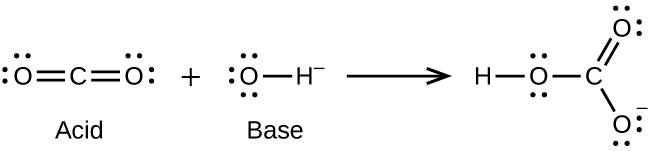;
(b)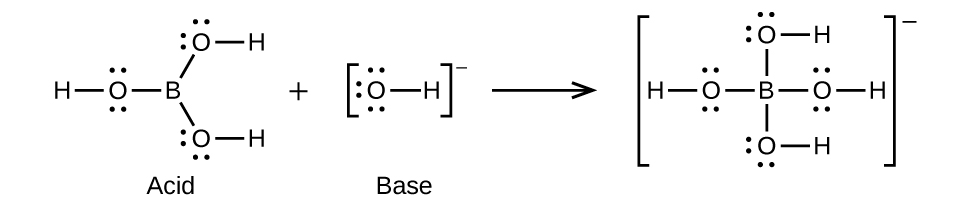;
(c)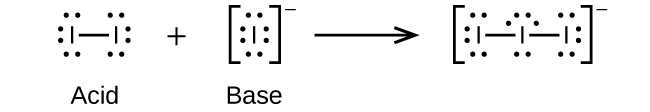;
(d)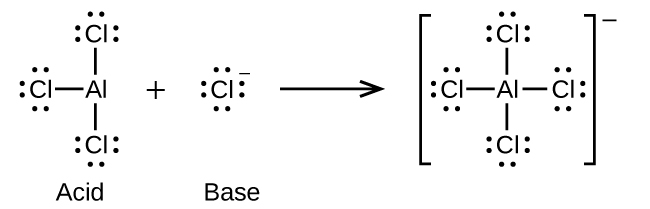;
(e)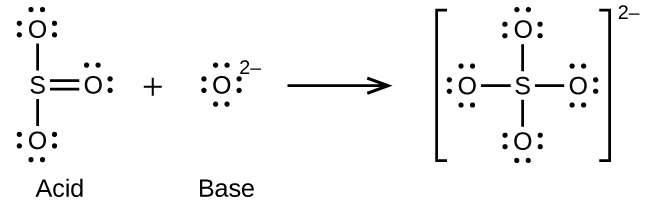Write the Lewis structures of the reactants and product of each of the following equations, and identify the Lewis acid and the Lewis base in each:

(a) ${\text{CS}}_{2}+{\text{SH}}^{\text{−}}\phantom{\rule{0.2em}{0ex}}⟶\phantom{\rule{0.2em}{0ex}}{\text{HCS}}_{3}{}^{\text{−}}$

(b) ${\text{BF}}_{3}+{\text{F}}^{\text{−}}\phantom{\rule{0.2em}{0ex}}⟶\phantom{\rule{0.2em}{0ex}}{\text{BF}}_{4}{}^{\text{−}}$

(c) ${\text{I}}^{\text{−}}+{\text{SnI}}_{2}\phantom{\rule{0.2em}{0ex}}⟶\phantom{\rule{0.2em}{0ex}}{\text{SnI}}_{3}{}^{\text{−}}$

(d) $\text{Al}{\left(\text{OH}\right)}_{3}+{\text{OH}}^{\text{−}}\phantom{\rule{0.2em}{0ex}}⟶\phantom{\rule{0.2em}{0ex}}\text{Al}{\left(\text{OH}\right)}_{4}{}^{\text{−}}$

(e) ${\text{F}}^{\text{−}}+{\text{SO}}_{3}\phantom{\rule{0.2em}{0ex}}⟶\phantom{\rule{0.2em}{0ex}}{\text{SFO}}_{3}{}^{\text{−}}$

Using Lewis structures, write balanced equations for the following reactions:

(a) $\text{HCl}\left(g\right)+{\text{PH}}_{3}\left(g\right)\phantom{\rule{0.2em}{0ex}}⟶\phantom{\rule{0.2em}{0ex}}$

(b) ${\text{H}}_{3}{\text{O}}^{+}+{\text{CH}}_{3}{}^{\text{−}}\phantom{\rule{0.2em}{0ex}}⟶\phantom{\rule{0.2em}{0ex}}$

(c) $\text{CaO}+{\text{SO}}_{3}\phantom{\rule{0.2em}{0ex}}⟶\phantom{\rule{0.2em}{0ex}}$

(d) ${\text{NH}}_{4}{}^{+}+{\text{C}}_{2}{\text{H}}_{5}{\text{O}}^{\text{−}}\phantom{\rule{0.2em}{0ex}}⟶\phantom{\rule{0.2em}{0ex}}$

(a)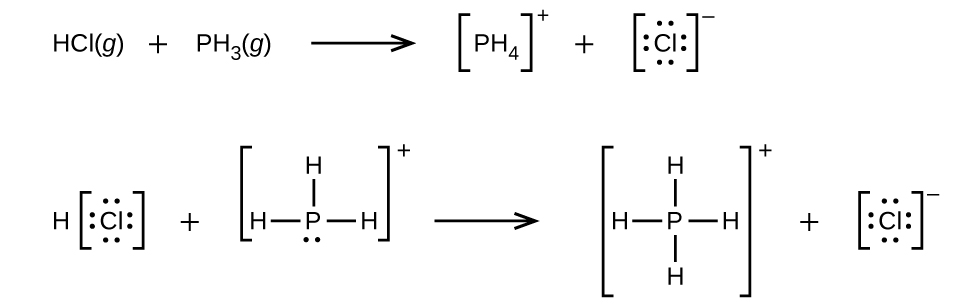;
(b) ${\text{H}}_{3}{\text{O}}^{+}+{\text{CH}}_{3}{}^{\text{−}}\phantom{\rule{0.2em}{0ex}}⟶\phantom{\rule{0.2em}{0ex}}{\text{CH}}_{4}+{\text{H}}_{2}\text{O}$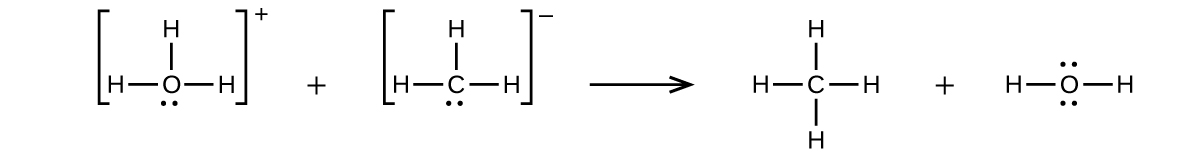;
(c) $\text{CaO}+{\text{SO}}_{3}\phantom{\rule{0.2em}{0ex}}⟶\phantom{\rule{0.2em}{0ex}}\text{CaSO}4$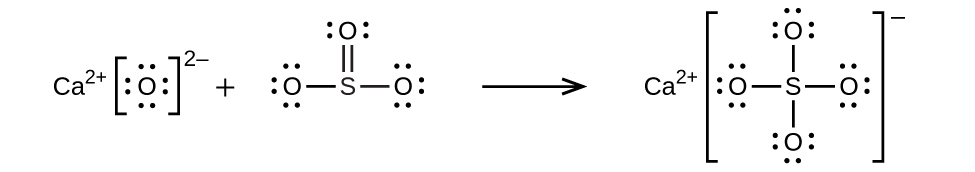;
(d) ${\text{NH}}_{4}{}^{+}+{\text{C}}_{2}{\text{H}}_{5}{\text{O}}^{\text{−}}\phantom{\rule{0.2em}{0ex}}⟶\phantom{\rule{0.2em}{0ex}}{\text{C}}_{2}{\text{H}}_{5}\text{OH}+{\text{NH}}_{3}$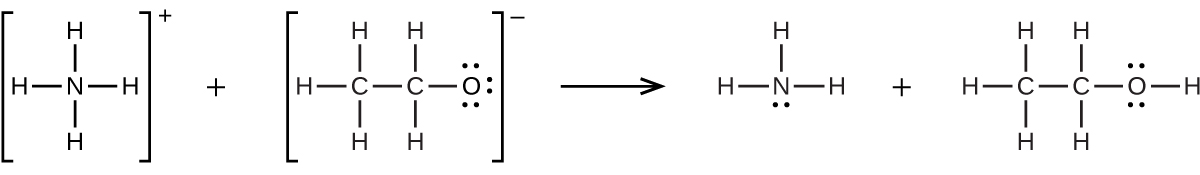Calculate $\left[{\text{HgCl}}_{4}{}^{\text{2−}}\right]$ in a solution prepared by adding 0.0200 mol of NaCl to 0.250 L of a 0.100- M HgCl 2 solution.

In a titration of cyanide ion, 28.72 mL of 0.0100 M AgNO 3 is added before precipitation begins. [The reaction of Ag + with CN goes to completion, producing the $\text{Ag}{\left(\text{CN}\right)}_{2}{}^{\text{−}}$ complex.] Precipitation of solid AgCN takes place when excess Ag + is added to the solution, above the amount needed to complete the formation of $\text{Ag}{\left(\text{CN}\right)}_{2}{}^{\text{−}}.$ How many grams of NaCN were in the original sample?

0.0281 g

What are the concentrations of Ag + , CN , and $\text{Ag}{\left(\text{CN}\right)}_{2}{}^{\text{−}}$ in a saturated solution of AgCN?

In dilute aqueous solution HF acts as a weak acid. However, pure liquid HF (boiling point = 19.5 °C) is a strong acid. In liquid HF, HNO 3 acts like a base and accepts protons. The acidity of liquid HF can be increased by adding one of several inorganic fluorides that are Lewis acids and accept F ion (for example, BF 3 or SbF 5 ). Write balanced chemical equations for the reaction of pure HNO 3 with pure HF and of pure HF with BF 3 .

${\text{HNO}}_{3}\left(l\right)+\text{HF}\left(l\right)\phantom{\rule{0.2em}{0ex}}⟶\phantom{\rule{0.2em}{0ex}}{\text{H}}_{2}{\text{NO}}_{3}{}^{+}+{\text{F}}^{\text{−}};$ $\text{HF}\left(l\right)+{\text{BF}}_{3}\left(g\right)\phantom{\rule{0.2em}{0ex}}⟶\phantom{\rule{0.2em}{0ex}}{\text{H}}^{+}+{\text{BF}}_{4}$

The simplest amino acid is glycine, H 2 NCH 2 CO 2 H. The common feature of amino acids is that they contain the functional groups: an amine group, –NH 2 , and a carboxylic acid group, –CO 2 H. An amino acid can function as either an acid or a base. For glycine, the acid strength of the carboxyl group is about the same as that of acetic acid, CH 3 CO 2 H, and the base strength of the amino group is slightly greater than that of ammonia, NH 3 .

(a) Write the Lewis structures of the ions that form when glycine is dissolved in 1 M HCl and in 1 M KOH.

(b) Write the Lewis structure of glycine when this amino acid is dissolved in water. (Hint: Consider the relative base strengths of the –NH 2 and $-{\text{CO}}_{2}{}^{\text{−}}$ groups.)

Boric acid, H 3 BO 3 , is not a Brønsted-Lowry acid but a Lewis acid.

(a) Write an equation for its reaction with water.

(b) Predict the shape of the anion thus formed.

(c) What is the hybridization on the boron consistent with the shape you have predicted?

(a) ${\text{H}}_{3}{\text{BO}}_{3}+{\text{H}}_{2}\text{O}\phantom{\rule{0.2em}{0ex}}⟶\phantom{\rule{0.2em}{0ex}}{\text{H}}_{4}{\text{BO}}_{4}{}^{\text{−}}+{\text{H}}^{+};$ (b) The electronic and molecular shapes are the same—both tetrahedral. (c) The tetrahedral structure is consistent with sp 3 hybridization.

what are oxidation numbers
pls what is electrolysis
Electrolysis is the process by which ionic substances are decomposed (broken down) into simpler substances when an electric current is passed through them. ... Electricity is the flow of electrons or ions. For electrolysis to work, the compound must contain ions.
AZEEZ
thanks
Idowu
what is the basicity of an atom
basicity is the number of replaceable Hydrogen atoms in a Molecule. in H2SO4, the basicity is 2. in Hcl, the basicity is 1
Inemesit
how to solve oxidation number
mention some examples of ester
do you mean ether?
Megan
what do converging lines on a mass Spectra represent
would I do to help me know this topic ?
Bulus
oi
Amargo
what the physic?
who is albert heistein?
Bassidi
similarities between elements in the same group and period
what is the ratio of hydrogen to oxulygen in carbohydrates
bunubyyvyhinuvgtvbjnjnygtcrc
yvcrzezalakhhehuzhbshsunakakoaak
what is poh and ph
please what is the chemical configuration of sodium
Sharon
2.8.1
david
1s²2s²2p⁶3s¹
Haile
2, 6, 2, 1
Salman
1s2, 2s2, 2px2, 2py2, 2pz2, 3s1
Justice
1s2,2s2,2py2,2
Maryify
1s2,2s2,2p6,
Francis
1s2,2s2,2px2,2py2,2pz2,3s1
Nnyila
what is criteria purity
cathode is a negative ion why is it that u said is negative
cathode is a negative electrode while cation is a positive ion. cation move towards cathode plate.
king
CH3COOH +NaOH ,complete the equation
compare and contrast the electrical conductivity of HCl and CH3cooH
The must be in dissolved in water (aqueous). Electrical conductivity is measured in Siemens (s). HCl (aq) has higher conductivity, as it fully ionises (small portion of CH3COOH (aq) ionises) when dissolved in water. Thus, more free ions to carry charge.
Abdelkarim
HCl being an strong acid will fully ionize in water thus producing more mobile ions for electrical conduction than the carboxylic acid
Valentine
differiante between a weak and a strong acid
david
how can I tell when an acid is weak or Strong
Amarachi
an aqueous solution of copper sulphate was electrolysed between graphite electrodes. state what was observed at the cathode
write the equation for the reaction that took place at the anode
Bakanya
what is enthalpy of combustion
Bakanya
Enthalpy change of combustion: It is the enthalpy change when 1 mole of substance is combusted with excess oxygen under standard conditions. Elements are in their standard states. Conditions: pressure = 1 atm Temperature =25°C
Abdelkarim
Observation at Cathode: Cu metal deposit (pink/red solid).
Abdelkarim
Equation at Anode: (SO4)^2- + 4H^+ + 2e^- __> SO2 + 2H2O
Abdelkarim
Equation : CuSO4 -> Cu^2+ + SO4^2- equation at katode: 2Cu^2+ + 4e -> 2Cu equation at anode: 2H2O -> 4H+ + O2 +4e at the anode which reacts is water because SO4 ^ 2- cannot be electrolyzed in the anode
NikenByByBy Janet ForresterBy Brooke DelaneyBy Brooke DelaneyBy JavaChamp TeamBy Madison ChristianBy Edward BitonBy Richley CrapoBy RhodesBy OpenStaxBy Naveen Tomar